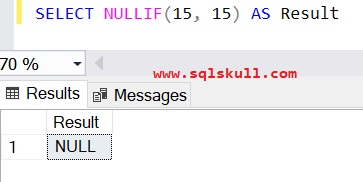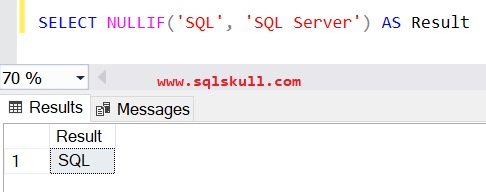Home » SQL NULLIF

# SQL NULLIFSQL NULLIF expression compares two expression and returns a null value if the two specified expressions are equal, else it returns the first expression.

NULLIF  expression is equivalent to a searched CASE expression in which the two expressions are equal and the resulting expression is NULL.

For example:

The NULLIF(@val1, @val2) expression is equivalent to below case expression:

CASE
WHEN @val1=@val2 THEN NULL
ELSE @val1 END

SYNTAX

`NULLIF ( expression1 , expression2)`

expression1, expression2

is the scalar expressions, that will be compared and the values of the expressions must be of the same datatype.

Returns the same type as the first expression1.

Lets take some example of using NULLIF in SQL Server.

#### Using NULLIF with integer values

Following statement returns the first value because first and second argument values are not equal.

`SELECT NULLIF(15, 1) AS Result`Following statement returns null because the value of first argument is equal to the value of second argument.

`SELECT NULLIF(15, 15) AS Result`#### Using NULLIF with string values

Following statement returns the first value because first and second argument values are not equal.

`SELECT NULLIF('SQL', 'SQL Server') AS Result`Following statement returns null because the value of first argument is equal to the value of second argument.#### NULLIF expression is equivalent to Searched CASE Statement

NULLIF expression is equivalent to a searched CASE expression in which the two expressions are equal and the resulting expression is NULL.

The NULLIF(@val1, @val2) expression is equivalent to below case expression:

CASE
WHEN @val1=@val2 THEN NULL
ELSE @val1 END

Lets take a look at following example.

```DECLARE @Val1 INT = 15, @Val2 INT = 1;

SELECT
NULLIF(@Val1,@Val2) AS Result_1;

SELECT CASE
WHEN @val1=@val2 THEN NULL
ELSE @val1 END AS Result_2 ;

GO```

You can see, the NULLIFF expression returns the first value that is 15 because first and second argument values are not equal. Also case expression returns 15.